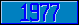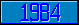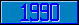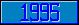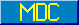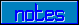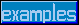## \$%MTXMUL

### M[UMPS] by Example

```
2-Jul–96, 6:54:35

Routine Save for Matrix Mathematics Library function \$%MTXMUL^MATH

;

; Unless otherwise noted, the code below

; was approved in document X11/96–26

;

; If corrections have been applied,

; first the original line appears,

; with three semicolons at the beginning of the line.

;

; Then the source of the correction is acknowledged,

; then the corrected line appears, followed by a

; line containing three semicolons.

;

MTXMUL(A,B,R,M,L,N) ;

; Multiply A[M,L] by B[L,N], result goes to R[M,N]

IF \$DATA(A)<10 QUIT 0

IF \$DATA(B)<10 QUIT 0

IF \$GET(L)<1 QUIT 0

IF \$GET(M)<1 QUIT 0

IF \$GET(N)<1 QUIT 0

;

NEW I,J,K,SUM,ANY

FOR I=1:1:M FOR J=1:1:N DO

. SET (SUM,ANY)=0

. KVALUE R(I,J)

. FOR K=1:1:L DO

. . SET:\$DATA(A(I,K))#2 ANY=1

. . SET:\$DATA(B(K,J))#2 ANY=1

. . SET SUM=\$GET(A(I,K))*\$GET(B(K,J))+SUM

. . QUIT

. SET:ANY R(I,J)=SUM

. QUIT

QUIT 1

;===

;

;

;

```